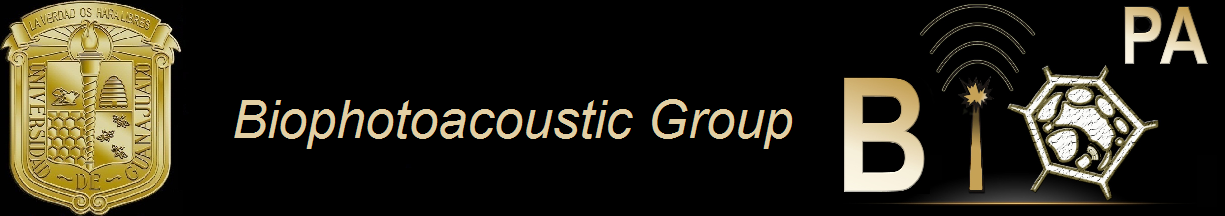Main Lab CV Summary CV.pdf (in Spanish) CV.docx (in Spanish) Research Introduction Research Projects Research Products Peer-reviewed Articles Articlesin extenso Citations to my articles Patents Collaborators Alumni Thesis Interest Homepages Contact Address:Loma del Bosque 103, Lomas del Campestre, Zip Code: 37150, Leon, Gto., Mexico. PO BOX. E-143. e-mail:ggutj@fisica.ugto.mx \begin{eqnarray} \left(\nabla^2-\frac{1}{\chi}\frac{\partial}{\partial t} \right) T(\mathbf{r}, t)&=&-\frac{1}{\kappa}H(\mathbf{r}, t),\\\\ \left(\nabla^2-\frac{1}{c^2}\frac{\partial^2}{\partial t^2}\right)p\left( \mathbf{r}, t \right)&=&-\rho\beta\frac{\partial^2}{\partial t^2}T\left(\mathbf{r}, t\right). \end{eqnarray} These are the Phenomena of Springs and springy bodies, which as they have not hitherto been by any that I know reduced to Rules, so have all the attempts for the explications of the reason of their power, and of springiness in general, been very insufficient. Robert Hooke, De Potentia Restitutiva (1678)